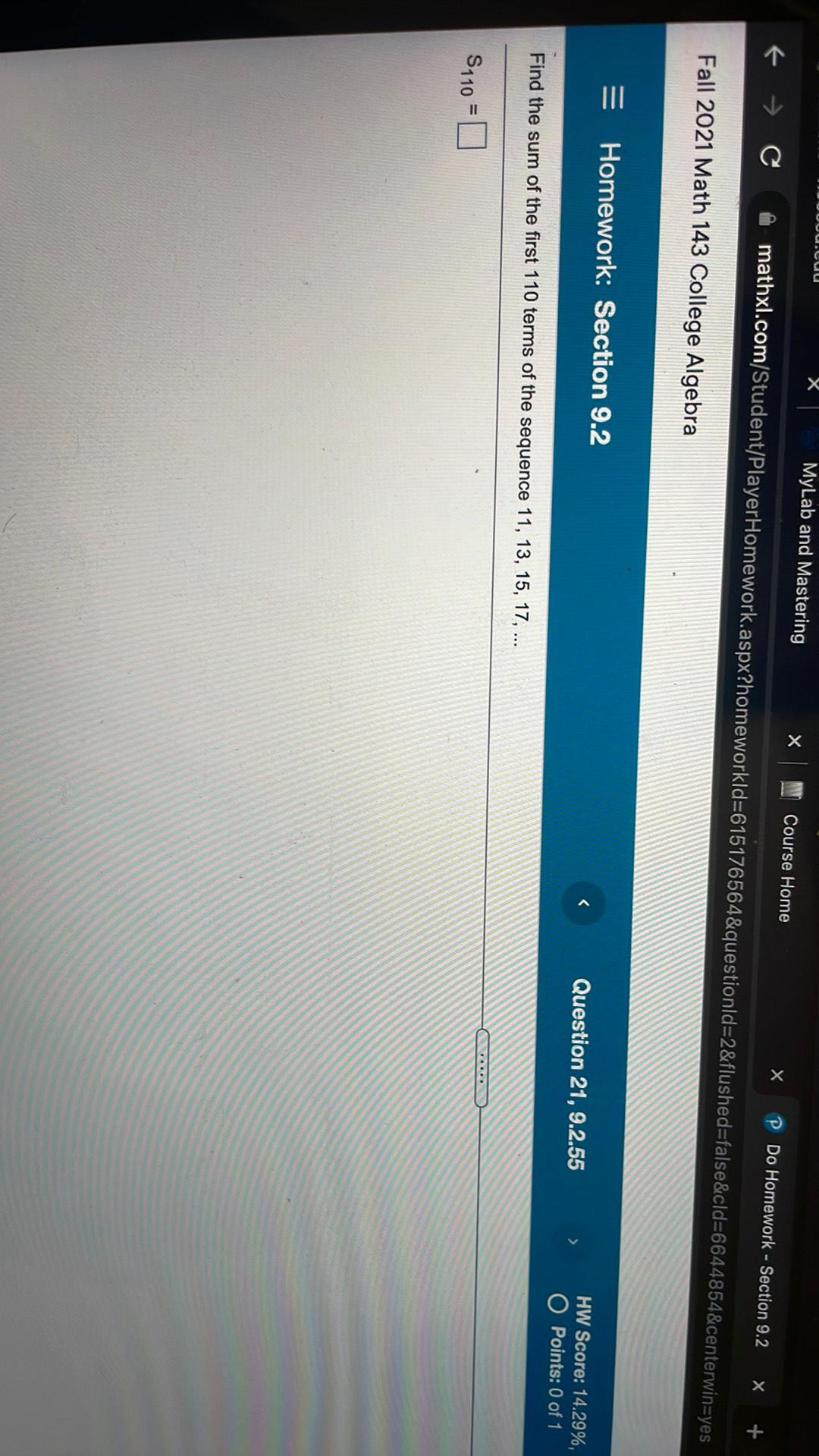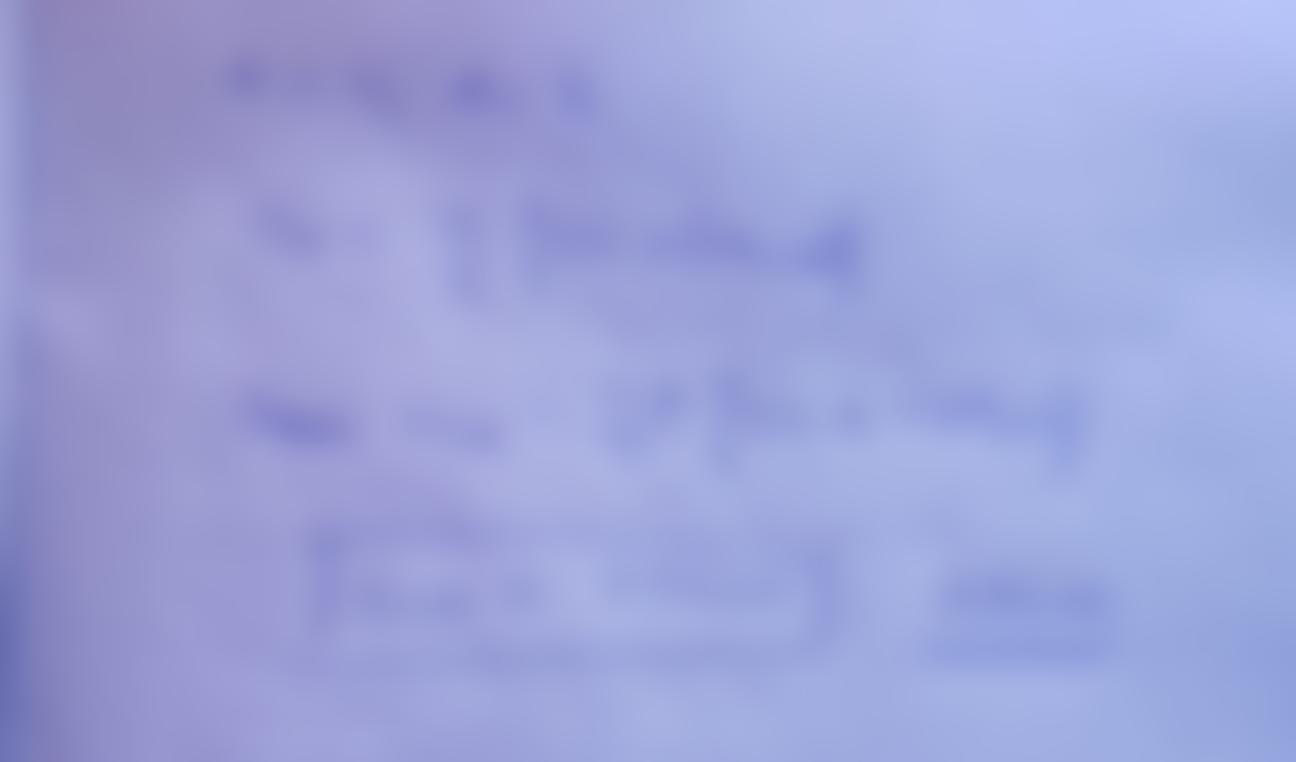Question:

JUUUU.au X MyLab and Mastering X Course Home € → C x mathxl.com/Student/PlayerHomework.aspx?homeworkld=615176564&questionld=2&flJUUUU.au X MyLab and Mastering X Course Home € → C x mathxl.com/Student/PlayerHomework.aspx?homeworkld=615176564&questionld=2&flushed=false&cld=6644854&centerwin=yes Do Homework - Section 9.2 X + Fall 2021 Math 143 College Algebra = Homework: Section 9.2 Question 21, 9.2.55 HW Score: 14.29%, O Points: 0 of 1 Find the sum of the first 110 terms of the sequence 11, 13, 15, 17, ... ..... \$110 =